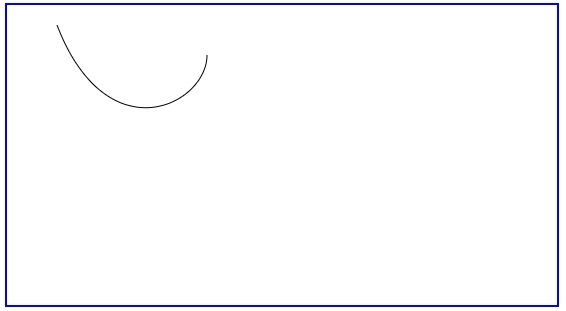# HTML Canvas to draw Bezier Curve

To draw a Bezier curve, use the BezierCurveTo() method in HTML. Let us first see the syntax −

ctx.bezierCurveTo(cp1x,cp1y,cp2x,cp2y,x,y);

Here,

• cp1x − Represents the x-coordinate of the first Bezier control point
• cp1y − Represents the y-coordinate of the first Bezier control point
• cp2x − Represents the x-coordinate of the second Bezier control point
• cp2y − Represents the y-coordinate of the second Bexier control point
• x − Represents the x-coordinate of the ending point
• y − Represents the y-coordinate of the ending point

Following is an example −

## Example

<!DOCTYPE html>
<html>
<title>HTML5 Canvas Tag</title>
<body>
<canvas id = "newCanvas" width = "550" height = "300" style = "border −2px solid blue;"></canvas>
<script>
var c = document.getElementById('newCanvas');
var ctx = c.getContext('2d');
ctx.beginPath();
ctx.moveTo(50, 20);
ctx.bezierCurveTo(100, 150, 200, 100, 200, 50);
ctx.stroke();
</script>
</body>
</html>

## Output